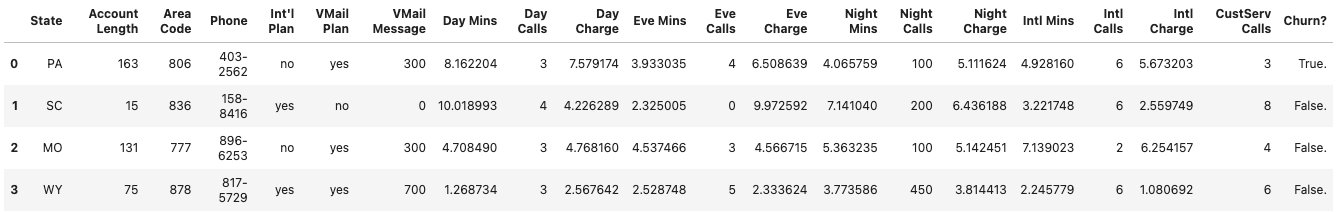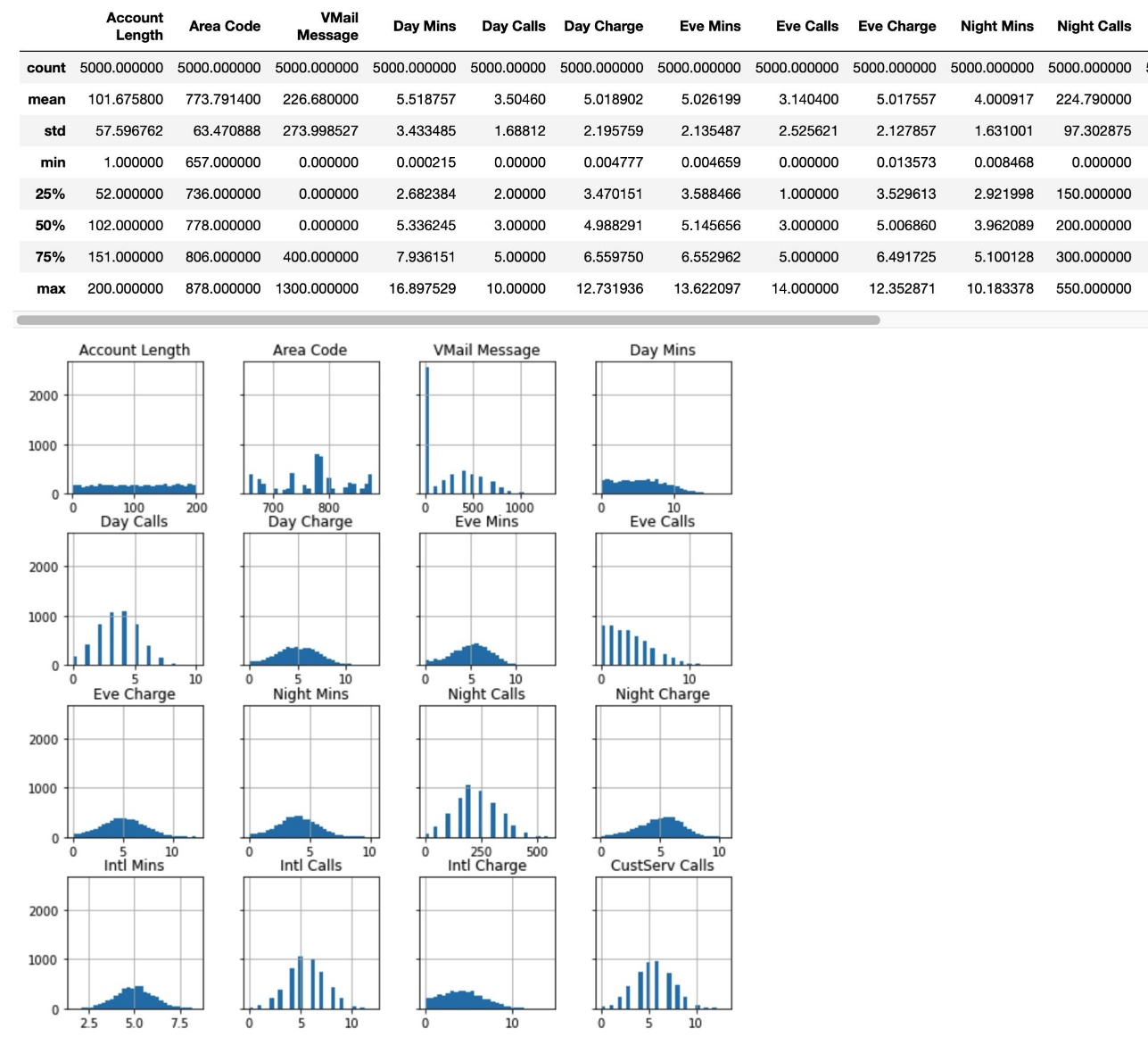# Churn prediction using Amazon SageMaker built-in tabular algorithms LightGBM, CatBoost, TabTransformer, and AutoGluon-Tabular

Amazon SageMaker provides a suite of built-in algorithms, pre-trained models, and pre-built solution templates to help data scientists and machine learning (ML) practitioners get started on training and deploying ML models quickly. These algorithms and models can be used for both supervised and unsupervised learning. They can process various types of input data, including tabular, image, and text.

Customer churn is a problem faced by a wide range of companies, from telecommunications to banking, where customers are typically lost to competitors. It’s in a company’s best interest to retain existing customers rather than acquire new customers because it usually costs significantly more to attract new customers. Mobile operators have historical records in which customers continued using the service or ultimately ended up churning. We can use this historical information of a mobile operator’s churn to train an ML model. After training this model, we can pass the profile information of an arbitrary customer (the same profile information that we used to train the model) to the model, and have it predict whether this customer is going to churn or not.

In this post, we train and deploy four recently released SageMaker algorithms—LightGBM, CatBoost, TabTransformer, and AutoGluon-Tabular—on a churn prediction dataset. We use SageMaker Automatic Model Tuning (a tool for hyperparameter optimization) to find the best hyperparameters for each model, and compare their performance on a holdout test dataset to select the optimal one.

You can also use this solution as a template to search over a collection of state-of-the-art tabular algorithms and use hyperparameter optimization to find the best overall model. You can easily replace the example dataset with your own to solve real business problems you’re interested in. If you want to jump straight into the SageMaker SDK code we go through in this post, you can refer to the following sample Jupyter notebook.

## Benefits of SageMaker built-in algorithms

When selecting an algorithm for your particular type of problem and data, using a SageMaker built-in algorithm is the easiest option, because doing so comes with the following major benefits:

• Low coding – The built-in algorithms require little coding to start running experiments. The only inputs you need to provide are the data, hyperparameters, and compute resources. This allows you to run experiments more quickly, with less overhead for tracking results and code changes.
• Efficient and scalable algorithm implementations – The built-in algorithms come with parallelization across multiple compute instances and GPU support right out of the box for all applicable algorithms. If you have a lot of data with which to train your model, most built-in algorithms can easily scale to meet the demand. Even if you already have a pre-trained model, it may still be easier to use its corollary in SageMaker and input the hyperparameters you already know rather than port it over and write a training script yourself.
• Transparency – You’re the owner of the resulting model artifacts. You can take that model and deploy it on SageMaker for several different inference patterns (check out all the available deployment types) and easy endpoint scaling and management, or you can deploy it wherever else you need it.

## Data visualization and preprocessing

First, we gather our customer churn dataset. It’s a relatively small dataset with 5,000 records, where each record uses 21 attributes to describe the profile of a customer of an unknown US mobile operator. The attributes range from the US state where the customer resides, to the number of calls they placed to customer service, to the cost they are billed for daytime calls. We’re trying to predict whether the customer will churn or not, which is a binary classification problem. The following is a subset of those features look like, with the label as the last column.The following are some insights for each column, specifically the summary statistics and histogram of selected features.We then preprocess the data, split it into training, validation, and test sets, and upload the data to Amazon Simple Storage Service (Amazon S3).

## Automatic model tuning of tabular algorithms

Hyperparameters control how our underlying algorithms operate and influence the performance of the model. Those hyperparameters can be the number of layers, learning rate, weight decay rate, and dropout for neural network-based models, or the number of leaves, iterations, and maximum tree depth for tree ensemble models. To select the best model, we apply SageMaker automatic model tuning to each of the four trained SageMaker tabular algorithms. You need only select the hyperparameters to tune and a range for each parameter to explore. For more information about automatic model tuning, refer to Amazon SageMaker Automatic Model Tuning: Using Machine Learning for Machine Learning or Amazon SageMaker automatic model tuning: Scalable gradient-free optimization.

Let’s see how this works in practice.

### LightGBM

We start by running automatic model tuning with LightGBM, and adapt that process to the other algorithms. As is explained in the post Amazon SageMaker JumpStart models and algorithms now available via API, the following artifacts are required to train a pre-built algorithm via the SageMaker SDK:

• Its framework-specific container image, containing all the required dependencies for training and inference
• The training and inference scripts for the selected model or algorithm

We first retrieve these artifacts, which depend on the `model_id` (`lightgbm-classification-model` in this case) and version:

``````from sagemaker import image_uris, model_uris, script_uris
train_model_id, train_model_version, train_scope = "lightgbm-classification-model", "*", "training"
training_instance_type = "ml.m5.4xlarge"

# Retrieve the docker image
train_image_uri = image_uris.retrieve(region=None,
framework=None,
model_id=train_model_id,
model_version=train_model_version,
image_scope=train_scope,
instance_type=training_instance_type,
)
# Retrieve the training script
train_source_uri = script_uris.retrieve(model_id=train_model_id,
model_version=train_model_version,
script_scope=train_scope
)
# Retrieve the pre-trained model tarball (in the case of tabular modeling, it is a dummy file)
train_model_uri = model_uris.retrieve(model_id=train_model_id,
model_version=train_model_version,
model_scope=train_scope)``````

We then get the default hyperparameters for LightGBM, set some of them to selected fixed values such as number of boosting rounds and evaluation metric on the validation data, and define the value ranges we want to search over for others. We use the SageMaker parameters `ContinuousParameter` and `IntegerParameter` for this:

``````from sagemaker import hyperparameters
from sagemaker.tuner import ContinuousParameter, IntegerParameter, HyperparameterTuner

# Retrieve the default hyper-parameters for fine-tuning the model
hyperparameters = hyperparameters.retrieve_default(model_id=train_model_id,
model_version=train_model_version
)
# [Optional] Override default hyperparameters with custom values
hyperparameters["num_boost_round"] = "500"
hyperparameters["metric"] = "auc"

# Define search ranges for other hyperparameters
hyperparameter_ranges_lgb = {
"learning_rate": ContinuousParameter(1e-4, 1, scaling_type="Logarithmic"),
"num_boost_round": IntegerParameter(2, 30),
"num_leaves": IntegerParameter(10, 50),
"feature_fraction": ContinuousParameter(0, 1),
"bagging_fraction": ContinuousParameter(0, 1),
"bagging_freq": IntegerParameter(1, 10),
"max_depth": IntegerParameter(5, 30),
"min_data_in_leaf": IntegerParameter(5, 50),
}``````

Finally, we create a SageMaker Estimator, feed it into a HyperarameterTuner, and start the hyperparameter tuning job with `tuner.fit()`:

``````from sagemaker.estimator import Estimator
from sagemaker.tuner import HyperParameterTuner

# Create SageMaker Estimator instance
tabular_estimator = Estimator(
role=aws_role,
image_uri=train_image_uri,
source_dir=train_source_uri,
model_uri=train_model_uri,
entry_point="transfer_learning.py",
instance_count=1,
instance_type=training_instance_type,
max_run=360000,
hyperparameters=hyperparameters,
)

tuner = HyperparameterTuner(
tabular_estimator,
"auc",
hyperparameter_ranges_lgb,
[{"Name": "auc", "Regex": "auc: ([0-9\.]+)"}],
max_jobs=10,
max_parallel_jobs=5,
objective_type="Maximize",
base_tuning_job_name="some_name",
)

tuner.fit({"training": training_dataset_s3_path}, logs=True)``````

The `max_jobs` parameter defines how many total jobs will be run in the automatic model tuning job, and `max_parallel_jobs` defines how many concurrent training jobs should be started. We also define the objective to `“Maximize”` the model’s AUC (area under the curve). To dive deeper into the available parameters exposed by `HyperParameterTuner`, refer to HyperparameterTuner.

Check out the sample notebook to see how we proceed to deploy and evaluate this model on the test set.

### CatBoost

The process for hyperparameter tuning on the CatBoost algorithm is the same as before, although we need to retrieve model artifacts under the ID `catboost-classification-model` and change the range selection of hyperparameters:

``````from sagemaker import hyperparameters

# Retrieve the default hyper-parameters for fine-tuning the model
hyperparameters = hyperparameters.retrieve_default(
model_id=train_model_id, model_version=train_model_version
)
# [Optional] Override default hyperparameters with custom values
hyperparameters["iterations"] = "500"
hyperparameters["eval_metric"] = "AUC"

# Define search ranges for other hyperparameters
hyperparameter_ranges_cat = {
"learning_rate": ContinuousParameter(0.00001, 0.1, scaling_type="Logarithmic"),
"iterations": IntegerParameter(50, 1000),
"depth": IntegerParameter(1, 10),
"l2_leaf_reg": IntegerParameter(1, 10),
"random_strength": ContinuousParameter(0.01, 10, scaling_type="Logarithmic"),
}``````

### TabTransformer

The process for hyperparameter tuning on the TabTransformer model is the same as before, although we need to retrieve model artifacts under the ID `pytorch-tabtransformerclassification-model` and change the range selection of hyperparameters.

We also change the training `instance_type` to `ml.p3.2xlarge`. TabTransformer is a model recently derived from Amazon research, which brings the power of deep learning to tabular data using Transformer models. To train this model in an efficient manner, we need a GPU-backed instance. For more information, refer to Bringing the power of deep learning to data in tables.

``````from sagemaker import hyperparameters
from sagemaker.tuner import CategoricalParameter

# Retrieve the default hyper-parameters for fine-tuning the model
hyperparameters = hyperparameters.retrieve_default(
model_id=train_model_id, model_version=train_model_version
)
# [Optional] Override default hyperparameters with custom values
hyperparameters["n_epochs"] = 40  # The same hyperparameter is named as "iterations" for CatBoost
hyperparameters["patience"] = 10

# Define search ranges for other hyperparameters
hyperparameter_ranges_tab = {
"learning_rate": ContinuousParameter(0.001, 0.01, scaling_type="Auto"),
"batch_size": CategoricalParameter([64, 128, 256, 512]),
"attn_dropout": ContinuousParameter(0.0, 0.8, scaling_type="Auto"),
"mlp_dropout": ContinuousParameter(0.0, 0.8, scaling_type="Auto"),
"input_dim": CategoricalParameter(["16", "32", "64", "128", "256"]),
"frac_shared_embed": ContinuousParameter(0.0, 0.5, scaling_type="Auto"),
}``````

### AutoGluon-Tabular

In the case of AutoGluon, we don’t run hyperparameter tuning. This is by design, because AutoGluon focuses on ensembling multiple models with sane choices of hyperparameters and stacking them in multiple layers. This ends up being more performant than training one model with the perfect selection of hyperparameters and is also computationally cheaper. For details, check out AutoGluon-Tabular: Robust and Accurate AutoML for Structured Data.

Therefore, we switch the `model_id` to `autogluon-classification-ensemble`, and only fix the evaluation metric hyperparameter to our desired AUC score:

``````from sagemaker import hyperparameters

# Retrieve the default hyper-parameters for fine-tuning the model
hyperparameters = hyperparameters.retrieve_default(
model_id=train_model_id, model_version=train_model_version
)

hyperparameters["eval_metric"] = "roc_auc"``````

Instead of calling `tuner.fit()`, we call `estimator.fit()` to start a single training job.

## Benchmarking the trained models

After we deploy all four models, we send the full test set to each endpoint for prediction and calculate accuracy, F1, and AUC metrics for each (see code in the sample notebook). We present the results in the following table, with an important disclaimer: results and relative performance between these models will depend on the dataset you use for training. These results are representative, and even though the tendency for certain algorithms to perform better is based on relevant factors (for example, AutoGluon intelligently ensembles the predictions of both LightGBM and CatBoost models behind the scenes), the balance in performance might change given a different data distribution.

 . LightGBM with Automatic Model Tuning CatBoost with Automatic Model Tuning TabTransformer with Automatic Model Tuning AutoGluon-Tabular Accuracy 0.8977 0.9622 0.9511 0.98 F1 0.8986 0.9624 0.9517 0.98 AUC 0.9629 0.9907 0.989 0.9979

## Conclusion

In this post, we trained four different SageMaker built-in algorithms to solve the customer churn prediction problem with low coding effort. We used SageMaker automatic model tuning to find the best hyperparameters to train these algorithms with, and compared their performance on a selected churn prediction dataset. You can use the related sample notebook as a template, replacing the dataset with your own to solve your desired tabular data-based problem.

Make sure to try these algorithms on SageMaker, and check out sample notebooks on how to use other built-in algorithms available on GitHub.Dr. Xin Huang is an Applied Scientist for Amazon SageMaker JumpStart and Amazon SageMaker built-in algorithms. He focuses on developing scalable machine learning algorithms. His research interests are in the area of natural language processing, explainable deep learning on tabular data, and robust analysis of non-parametric space-time clustering. He has published many papers in ACL, ICDM, KDD conferences, and Royal Statistical Society: Series A journal.João Moura is an AI/ML Specialist Solutions Architect at Amazon Web Services. He is mostly focused on NLP use-cases and helping customers optimize Deep Learning model training and deployment. He is also an active proponent of low-code ML solutions and ML-specialized hardware.